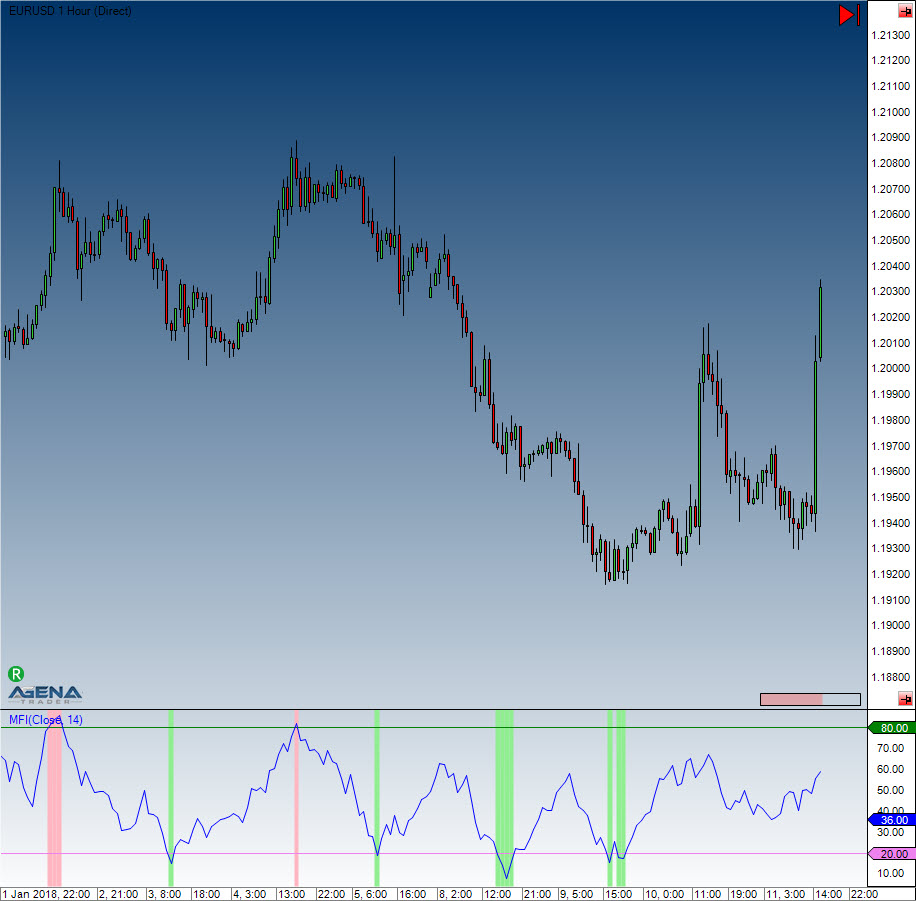Comment on page

# Money Flow Index (MFI)

#### Description

The MFI is an oscillator that makes use of both volume and price to measure buying and selling pressure. MFI, which was developed by Gene Quong and Avrum Soudack, is also known as volume-weighted RSI. MFI starts with the typical price for each period. Money flow is then positive when this aforementioned typical price increases (buying pressure) and negative when it decreases (selling pressure). A ratio of positive-negative money flow is then inserted into an RSI formula to come up with an oscillator that fluctuates between 0 and 100. The MFI, which is a momentum oscillator linked to volume, is best used for identifying reversals and price extremes with a variety of different signals.

#### Interpretation

The MFI is interpreted similarly to the RSI indicator. The MFI’s decisive factor is much stronger than the RSI’s when it comes to trend direction, due to the fact that volume is included in the calculation.

#### Usage

MFI(int period)
MFI(IDataSeries inSeries, int period)
MFI(int period)[int barsAgo]
MFI(IDataSeries inSeries, int period)[int barsAgo]

#### Return value

double
When using this method with an index (e.g. MFI(14)[int barsAgo] ), the value of the indicator will be issued for the referenced bar.

#### Parameters

inSeries Input data series for the indicator
period Number of bars included in the calculations

#### VisualizationMoney Flow Index (MFI)

#### Example

//Current MFI value
Print("The current value for the MFI is: " + MFI(14));